Question

A coil is formed by winding 190 turns of insulated 16 gauge copper wire (diameter = 1.9 mm) in a single layer on a cylindrical form of radius 9.2 cm. What is the resistance of the coil? Neglect the thickness of the insulation. (Take the resistivity of copper to be 1.69 × 10-8 ohm-m.)

1.MichaelMet

The resistance of the coil is 0.655 Ω

Explanation:

Given that,

Number of turns = 190 turns

Diameter of coil= 1.9 mm = 0.95 mm

Radius of single layer = 9.2 cm

Pressure = 16 gauge

We need to calculate the length of the wire

Using formula of length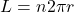Where, n = number of turns

Put the value into the formula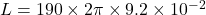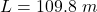We need to calculate the area of cross section

Using formula of area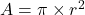Put the value into the formula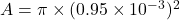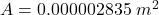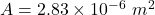We need to calculate the resistance of the coil

Using formula of resistivity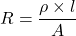Put the value into the formula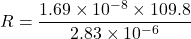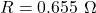Hence, The resistance of the coil is 0.655 Ω

2.Explanation:

The given data is as follows.

number of turns = n = 190 turns

radius = r = 9.2 cm = 0.092 m

diameter of copper wire = d = 1.9 mm

radius of copper wire =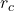=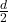=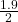=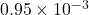m

where,  A = cross sectional area of the wire

As, length of each turn of wire is 2pr where r is radius of the coil.

L = 190(2pr)

=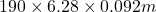= 109.77 m

The cross sectional area of the wire is as follows.

A =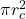=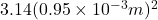=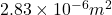It is given that resistivity of copper wire is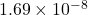R =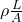=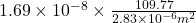=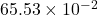Thus, we can conclude that resistance of the coil is.